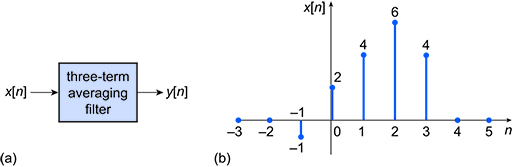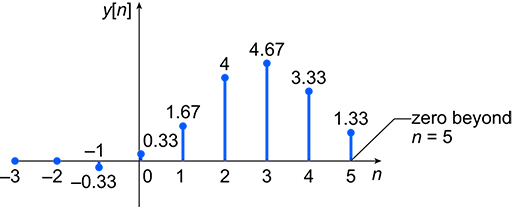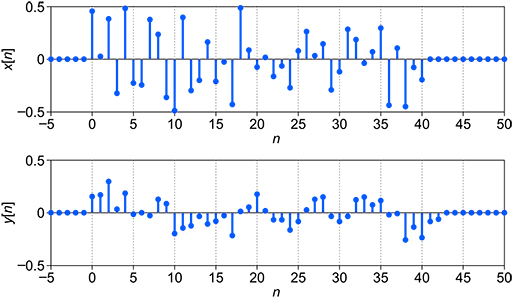Science, Maths & Technology

### Become an OU studentElectronic applications

Start this free course now. Just create an account and sign in. Enrol and complete the course for a free statement of participation or digital badge if available.

# 3.5 Digital filtering in the time domain

A simple form of digital filter is the three-term averaging filter, in which the output value is equal to the average of three successive signal sample values. In Figure 19(a), which shows a discrete-time signal applied to a digital three-term averaging filter, the output is given by

The signals and are spaced  seconds apart in time, where is the sampling interval. Similarly, and are spaced  seconds apart.

Figure 19(b) shows the values of the input signal that will be applied to the filter input.Figure 19 (a) Three-term averaging filter; (b) input signal to the three-term averaging filter

The values of and are listed in Table 1. Assume that for any and .

 n −3 −2 −1 0 1 2 3 4 5 x of n 0 0 −1 2 4 6 4 0 0

For the output to be calculated, the values of , and must be stored in memory and accessible to the processor performing the calculation. The order of a digital filter is the number of previous inputs (stored in the processor’s memory) used to calculate the current output, so this three-term averaging filter is second-order.

For values of the output will be 0, so the calculations below start at .

For you can substitute in values to give .

For you get .

For you get .

Table 2 shows the results of all calculations for , shown as decimal values to 2 significant figures. Note that you can stop at , since above this the output will be 0 again.

 n −3 −2 −1 0 1 2 3 4 5 x of n 0 0 −1 2 4 6 4 0 0 y of n 0 0 −0.33 0.33 1.67 4 4.67 3.33 1.33

The resulting output discrete-time waveform is given in Figure 20.Figure 20 Filter output in response to the input in Figure 19(b)

Figure 21 shows the same filter applied to an input that has more noise in the signal and a longer sequence.Figure 21 Longer sequence filtered by the three-term averaging filter (Wickert, 2011, p. 7)